# MeshSphere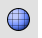Mesh

Polygon Mesh Primitives >

Sphere

The MeshSphere command draws a polygon mesh sphere.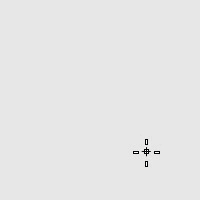Command-line options

Creates a sphere by picking the center point and a radius point.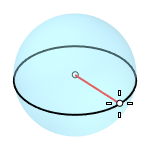##### 2Point

Creates a sphere from two opposite points on the base circle.##### 3Point

Creates a sphere from three points on the base circle.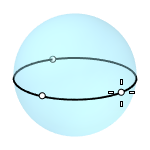##### Tangent

Creates a sphere with the base circle tangent to one, two or three curves.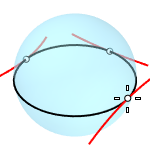##### AroundCurve

Creates a sphere from its center point on a curve, and a point on the base circle perpendicular to the curve.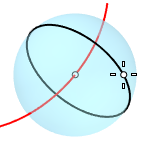##### 4Point

Creates a sphere from three points on a section circle and a point on the sphere.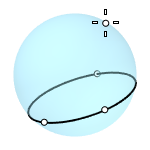##### FitPoints

Creates a sphere by fitting to selected point objects, curve and surface control points, and mesh vertices.### Styles##### UV

Creates a UV mesh sphere made out of quad faces and a triangle fan at the poles.

##### VerticalFaces

The face count in the vertical direction.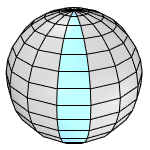##### AroundFaces

The face count in the horizontal direction.##### QuadsCreates a quad mesh sphere with the vertices evenly distributed. A quad mesh sphere starts from a cube (a regular polyhedron with 6 faces). It is then subdivided by the times specified in the Subdivisions option.

##### Subdivisions

Refines the quad mesh sphere by subdividing each quad into 4 smaller quads every time. The subdivision range is from 0 (6 faces) to 6 (24576 faces).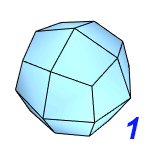##### TrianglesCreates an icospherical mesh with the vertices evenly distributed. A mesh icosphere starts from an icosahedron (a regular polyhedron with 20 equilateral triangles). It is then subdivided by the times specified in the Subdivisions option.

##### Subdivisions

Refines the icospherical mesh by subdividing each triangle into 4 smaller triangles every time. The subdivision range is from 0 (20 faces) to 5 (20480 faces).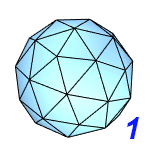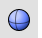##### Sphere

Draw a solid sphere.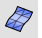##### Mesh

Create a mesh from a NURBS surface or polysurface.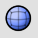##### SubDSphereCreates a subdivision sphere object.

Rhinoceros 7 © 2010-2023 Robert McNeel & Associates. 03-May-2023